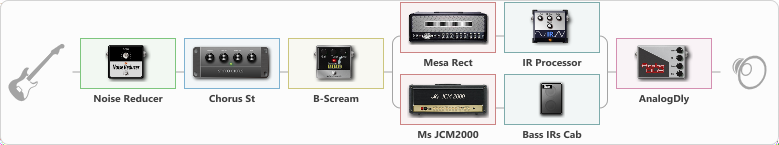# Charvel

Discussion in 'ToneLib-GFX presets' started by carlinos3, Feb 1, 2023.

1. Charvel
Preset name: Charvel

Effects chain:Effect: "Noise Reducer" (Dynamics / Filter), active - "yes"
{

"Sens" = 64
"Mode" = Hard
}

Effect: "Chorus St" (Modulation / Sfx), active - "yes"
{

"Speed" = 1.9
"Depth" = 31
"Center" = 4.4
"Mix" = 29
}

Effect: "B-Scream" (Overdrive / Distortion), active - "yes"
{

"Drive" = 67
"Tone" = 74
"Level" = 60
}

Effect: "Splitter" (Dynamics / Filter)
{

"A-Bypass" = Off
"A-Pan" = -25
"A-Level" = 55
"B-Bypass" = Off
"B-Pan" = 25
"B-Level" = 55
"Width" = 0
'A' branch:
{

Effect: "Mesa Rect" (Amp simulators), active - "yes"
{

"Gain" = 100
"Bass" = 50
"Middle" = 50
"Treble" = 50
"Presence" = 50
"Master" = 100
"Level (dB)" =0
}
Effect: "IR Processor" (Cabinets), active - "yes"
{

"IR" = HB_dc
"Low Cut (Hz)" = 0
"Hi Cut (kHz)" = 20.0
"Mix" = 100
"Level (dB)" = 7
}
}
'B' branch:
{

Effect: "Ms JCM2000" (Amp simulators), active - "yes"
{

"Gain" = 100
"Bass" = 50
"Middle" = 50
"Treble" = 50
"Presence" = 100
"Master" = 100
"Level (dB)" = 0
}
Effect: "Bass IRs Cab" (Cabinets), active - "yes"
{

"Model" = Hartke 4.5XL (4x10")
"Mic Position" = Middle
"Mic Distance" = Far
"Low Cut (Hz)" = 0
"Hi Cut (kHz)" = 20.0
"Mix" = 100
"Level (dB)" = 0
}
}
}

Effect: "AnalogDly" (Delay), active - "yes"
{

"Time" = 500
"Feedback" = 34
"Tone" = 65
"Mix" = 53
}

Note: You will need to download and install the ToneLib-GFX software to use the preset.

Last edited: Mar 7, 2023
dodori likes this.
2. Merci pour ce plug-in !